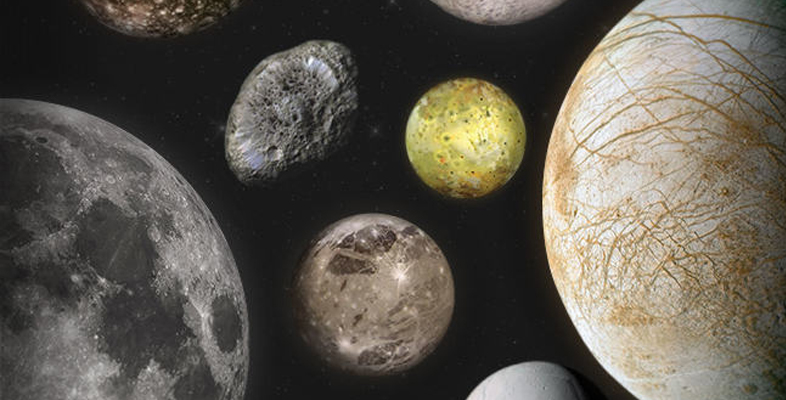Science, Maths & Technology

### Become an OU studentMoons of our Solar System

Start this free course now. Just create an account and sign in. Enrol and complete the course for a free statement of participation or digital badge if available.

# 1.3 How small? How expensive?

Now you have a chance to weigh up some of the costs of sending objects into space. It’s a very basic non-scored activity. Don’t worry if you don’t know some of the answers. Take an educated guess.

## Activity 1 The costs of sending objects into space

Timing: Allow approximately 15 minutes.

a.

$3000 b.$10 000

c.

$230 000 d.$600 000

b.

Well done.

a.

20 kg

b.

899 kg

c.

1000 kg

b.

Correct.

a.

$2 million b.$100 million

c.

$2.5 billion The correct answer is c. c. Correct. a.$10 billion

b.

$20 billion c.$150 billion

c.

Correct.

a.

$6 million b.$45 million

c.

$650 million d.$1 billion